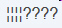3.NF.1.Test1B Understand fractions
 Name:    3.NF.1.Test1B Understand fractions

Multiple Choice
Identify the choice that best completes the statement or answers the question.

1.

What fraction of squares are shaded?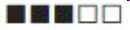a. 2/3 c. 3/5 b. 2/5 d. 5/5

2.

 a.c.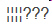b.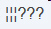d.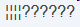3.

What fraction of squares is not shaded?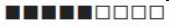a. 5/9 c. 4/5 b. 5/4 d. 4/9

4.

What fraction of squares is not shaded?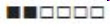a. 4/2 c. 4/6 b. 2/6 d. 2/4

5.

What fraction of circles are not shaded?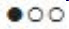a. 2/3 c. 1/3 b. 2/2 d. 1/2

6.

What fraction of circles are not shaded?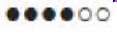a. 2/4 c. 4/6 b. 2/6 d. 4/2

7.

What fraction of circles are shaded?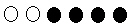a. 2/4 c. 2/6 b. 4/4 d. 4/6

8.

What fraction of circles are shaded?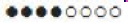a. 1/3 c. 4/4 b. 4/8 d. 8/4

9.

What fraction of squares are shaded?a. 3/7 c. 3/4 b. 4/7 d. 4/3

10.

 a.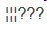c.b.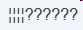d.